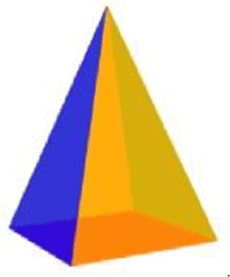Courses

# Test: Visualising Solid Shapes- 2

## 20 Questions MCQ Test Mathematics (Maths) Class 8 | Test: Visualising Solid Shapes- 2

Description
This mock test of Test: Visualising Solid Shapes- 2 for Class 8 helps you for every Class 8 entrance exam. This contains 20 Multiple Choice Questions for Class 8 Test: Visualising Solid Shapes- 2 (mcq) to study with solutions a complete question bank. The solved questions answers in this Test: Visualising Solid Shapes- 2 quiz give you a good mix of easy questions and tough questions. Class 8 students definitely take this Test: Visualising Solid Shapes- 2 exercise for a better result in the exam. You can find other Test: Visualising Solid Shapes- 2 extra questions, long questions & short questions for Class 8 on EduRev as well by searching above.
QUESTION: 1

Solution:
QUESTION: 2

### Find the side of a cube whose surface area is 2400 cm2.

Solution:

Surface area of  a cube = 6l2, where l is the length of its side
2400= 6l2
l= 2400/6
l2  = 400 = 202
l = 20 cm

QUESTION: 3

### Which of the statements is false for a pyramid?

Solution:
QUESTION: 4

Which of the statements is true?

Solution:

A square pyramid is a pyramid with a square base. So it has 4 triangular faces and 1 square base.

QUESTION: 5

Which of the following can be calculated only for a cone but not for a cylinder?

Solution:
QUESTION: 6

Which of the following statements is true?

Solution:
QUESTION: 7

Identify the statement that is false for a prism.

Solution:
QUESTION: 8

Identify the statement that is true.

Solution:
QUESTION: 9

The faces of a square prism consist of

Solution:
QUESTION: 10

A three dimensional shape is _________ object.

Solution:

Solid geometry is the traditional mane of the geometry of three-dimensional

QUESTION: 11

A square pyramid always has ___.

Solution:
QUESTION: 12

The faces of a triangular pyramid consist of

Solution:

There are 4 triangles in a triangular pyramid
A triangular pyramid is a pyramid with a triangle as a base and three triangular faces. It has four vertices or points and six edges.

QUESTION: 13

The faces of a pentagonal pyramid consist of

Solution:
QUESTION: 14

What is the name of this solid?Solution:
QUESTION: 15

Which is the three-dimensional figure formed by rotating a triangle?

Solution:
QUESTION: 16

The faces of a cube consist of

Solution:
QUESTION: 17

How many circular bases does a cylinder have?

Solution:

A cylinder is similar to a prism, but its two bases are circles, not polygons. Also the sides of a cylinder are curved, not flat.
There are 2 circular bases 1 on top and 1 on bottom.

QUESTION: 18

A cylinder has ____.

Solution:
QUESTION: 19

A hexagonal pyramid has ______.

Solution:
QUESTION: 20

An octagonal prism has ____.

Solution: